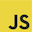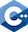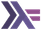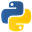CMSI 386
Final Exam Answers
1. x => y => x * yx -> y -> x * y[](double x){return [x](double y){return x * y;};}\x -> \y -> x * yAnother answer for Haskell is the three characters (*). Also, it was pretty common in C++ for people to forget the capture and the return keywords

1. You cannot write these in Haskell because there is no way to type the functions! For list to tuples, the input would have type List a but we can’t pick a a unique result type, since (a,a), (a,a,a,a,a,a), etc. are all different types—and Haskell does not have overloading. For tuples to lists, same reason, except we know the output type but cannot assign a unique input type.
2. toList3 :: (a,a,a) -> [a]
toList3 (x, y, z) = [x, y, z]2. function zip3({x, y, z}, [a, b, c]) {
return [[x, a], [y, b], [z, c]];
}Very important to pattern match both the object and the array. Pattern matching was the only acceptable answer here. Arrow functions were a nice try, but no.

3. Java’s designers think your functions are better when they are very simple and explicit: ALL parameters have a name and an explict type! Defaults and patterns in their mind just complicate things and make more things for you to learn, and are error-prone and hard for novices to read.

To simulate default parameters in Java, use overloading.

4. Is a null value a member of:
1. The JavaScript type "string"? Yes_________ No, but it's a member of ___Null_______
2. The Python type str? Yes_________ No, but it's a member of ______NoneType______
3. The Java type String? Yes__X_______ No, but it's a member of __________________________
4. The C++ type string? Yes_________ No, but it's a member of ______string*___
5. The Haskell type [Char]? Yes_________ No, but it's a member of ____Maybe [Char]______
5. Well the fact that that type error was detected at run time means Java is not 100% staticially typed. It is probably like 99% statically typed. You can say "almost completely statically typed" or "mostly statically typed." Just not "100% statically typed."
1. a = d; --okay, a dog is an animal
ap = &d; -- okay, an animal pointer can point to a dog
d = a; -- NO! a might be a different animal than a dog
dp = &a --NO! a might be a different animal than a dog
dp = (Dog*)(&a); --okay, you can cast explicitly, but beware
dp = (Dog*)cp; --okay, again, explicit cast are accepted by the compiler
ap = new Dog; --okay, an animal pointer can point to a dog
ap = &(new Cat) --NO! cannot take the address of an r-value
d = Dog(a) --NO! You cannot cast objects, only pointers.
2. No! Because animals.push_back(new Cat()) is and must always be allowed. So if we did allow animals = dogs then the compiler could not stop us from adding a cat to a list of dogs!
6. First, remember the question asked for an expression, not a function! Second, it’s true that the original problem from 1974 said return -1 if there was no such row, but modern languages have what Java and Swift call Optionals and what Haskell and Elm call Maybes. So let’s use those.
findIndex (all (==0)) aIntStream.range(0, a.length)
.filter(i -> Arrays.stream(a[i]).allMatch(x->x==0))
.findFirst()7. Here is the straightforward recursive solution (not tail-recursive):
function c(n) {
return n <= 1 ? 0 : 1 + c(n % 2 == 0 ? n / 2 : 3 * n + 1);
}def c(n):
return 0 if n <= 1 else 1 + c(n // 2 if n % 2 == 0 else 3 * n + 1)int c(int n) {
return n <= 1 ? 0 : 1 + c(n % 2 == 0 ? n / 2 : 3 * n + 1);
}c n | n <= 1 = 0 | otherwise = 1 + c (if even n then n div 2 else 3 * n + 1)8. Python requires that you implement inorder in both subclasses! In class Empty:
def inorder(self):
return []In class Node:
def inorder(self):
return self.left.inorder() + [self.data] + self.right.inorder()In Haskell you write the function on the top-level:

inorder :: BinaryTree a -> [a]
inorder Empty = []
inorder (Node data left right) = inorder left ++ [data] ++ inorder right1. You can’t move an L-value because an L-value is something that can be used later, and a move wipes out objects (leaves them all empty). R-values, OTOH, can not be used later so there is no problem pilfering their resources.
2. Moves exist because they are sooooooo efficient! They save the trouble of making multiple unncessary copies of possibly large objects. No copies are needed: just a few pointer moves is all it takes to do a move.